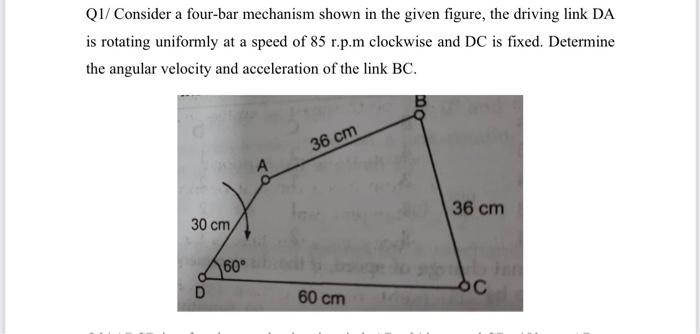# Consider a four-bar mechanism shown in the given figure, the driving link DA is rotating uniformly at a speed of 85 r.p.m clockwise and DC is fixed. Determine the angular velocity and acceleration of the link BC

Question-AnswerCategory: Kinematics of MachineConsider a four-bar mechanism shown in the given figure, the driving link DA is rotating uniformly at a speed of 85 r.p.m clockwise and DC is fixed. Determine the angular velocity and acceleration of the link BCConsider a four-bar mechanism shown in the given figure, the driving link DA is rotating uniformly at a speed of 85 r.p.m clockwise and DC is fixed. Determine the angular velocity and acceleration of the link BC